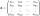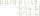# Matrix - problems

1. The inverseThe inverse matrix for matrix A has a determinant value of 0.333. What value has a determinant of the matrix A?
2. The determinantThe determinant of the unit matrix equals 7. Check how many rows the A matrix contains.
3. Inverse matrixFind how many times is the larger determinant is the matrix A, which equals 9 as the determinant of its inverse matrix.
4. Inverse matrixFind out inverse by Gauss elimination or by reduction method. A=[2/3. 1 -3. 1/3]

We apologize, but in this category are not a lot of examples.
Do you have an interesting mathematical problem that you can't solve it? Enter it, and we can try to solve it.

To this e-mail address, we will reply solution; solved examples are also published here. Please enter e-mail correctly and check whether you don't have a full mailbox.